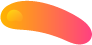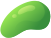##### binary calculations
1. Given the following assembly code complete each of the binary calculations by hand. Indicate the resulting value as a signed integer, the overflow flag and whether the result is valid as a signed integer.

mov al, 107 add al, 21
mov bl, -30 add bl, -98
(2) Write an assembly program that contains the following integer definitions:
val1: an 8-bit integer initialized to 23

val2: a 32-bit signed integer initialized to -117

val3: a 16-bit signed integer initialized to 62

The program should perform the calculation ((val3 – 1) – val2) – (val1 + 5) and save the result in a memory location named val4. You need only show the .code and .data segments of the code.

3.

(3) Write an assembly program that subtracts 5 from every value in an array. For example, if the array was (1,2,3,4,5) it should be changed to (-4,-3,-2,-1,0). Make the program as flexible as possible if the array size and type should be changed in the future.
You need only show the .code and .data segments of the code. a) Using indirect addressing.